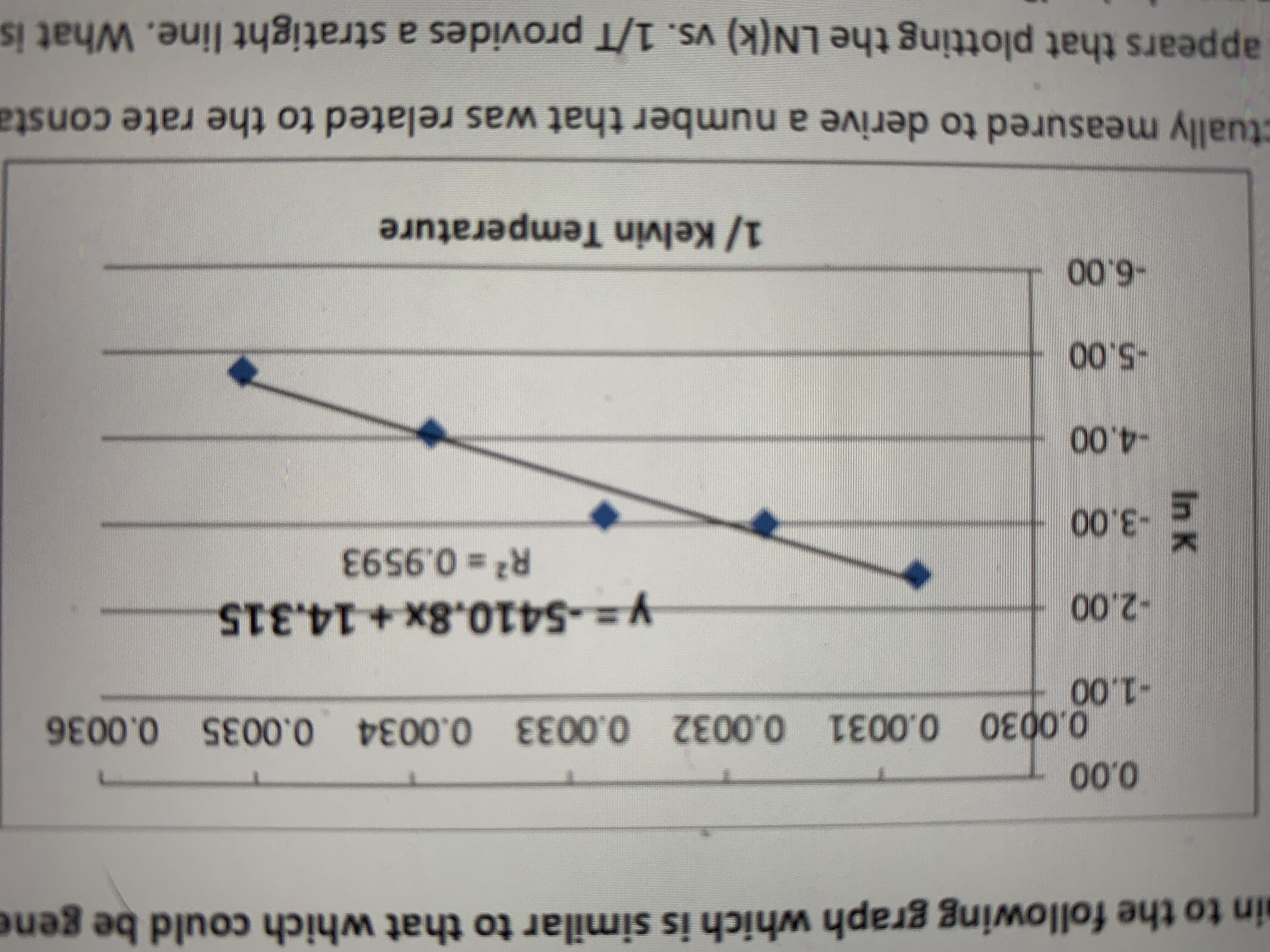# 19. What value was actually measured to derive a number that was related to the rate constant? 20. From the graph, it appears that plotting the LN(k) vs. 1/T provides a stratight line. What is the equation from which this relationship was derived? 21. Calculate the value for the energy of activation from the data provided. 22. What is the expected result on the rate of the reaction if the temperature is increased? 23. How was the “end” of the reaction signaled in the reaction as performed in the